This is rather a broad concept which is not only related to Math but also extends to Physics as well.

Procedure: For any two curvesand, its intersection is defined as the points where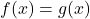. Of course,andare in terms of x. So, when we solve this equation, we get the values of x. Then, we plug it again inor(Doesn’t matter which one. Why?)  to get corresponding y coordinate. Hence, we get those point(s).

Here’s a worked-out example between a parabola and a straight line:

Different perspective: So. Let’s rewrite it as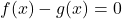. Let’s call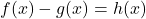.

So the equation becomes. Although solving this will again give us the same set(s) of coordinates which we found earlier. But this represents the “roots” or “solutions” of.

E.g.1: Height of two balls thrown is given byand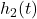where t represents time. Find the time when they are at same height?

So, we setup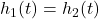and solve for t.

E.g.1 (rephrased): Height of two balls thrown is given byandwhere t represents time. Find the time when the difference between their height is 0?

So, we setup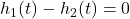and solve for t to get the same answers as above.

This concept encompasses other function types like Logarithms, Trigonometric etcetera as well.

E.g.2: Find the solutions ofin [0,2π]

When we solve this graphically, we plotand y=0.5 on the same set of coordinate axis and find its point of intersection.

While algebraically, we use trigonometric identities and properties (of sine in this case) and unit circle to find the values of x. I will leave this one as an exercise. If you get the final answers as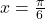and, you are on the right track!